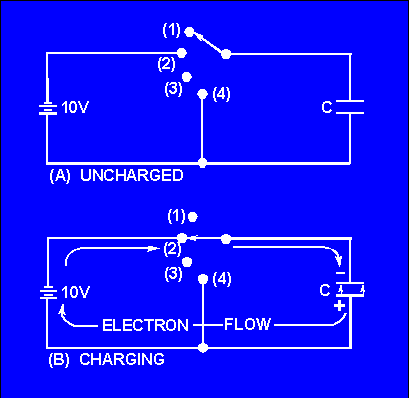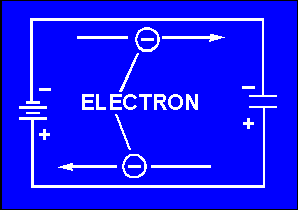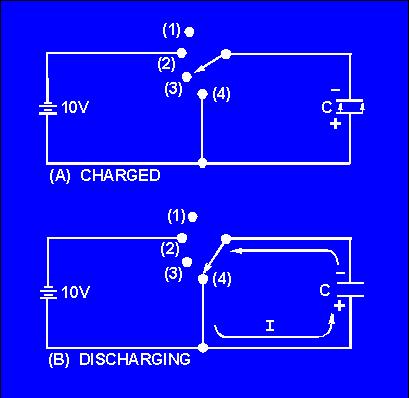Charging and Discharging a Capacitor internal resistance), although this is impossible in practice.">Custom SearchCHARGING AND DISCHARGING A CAPACITOR CHARGING In order to better understand the action of a capacitor in conjunction with other components, the charge and discharge actions of a purely capacitive circuit are analyzed first. For ease of explanation the capacitor and voltage source shown in figure 3-6 are assumed to be perfect (no internal resistance), although this is impossible in practice. In figure 3-6(A), an uncharged capacitor is shown connected to a four-position switch. With the switch in position 1 the circuit is open and no voltage is applied to the capacitor. Initially each plate of the capacitor is a neutral body and until a difference of potential is impressed across the capacitor, no electrostatic field can exist between the plates. Figure 3-6. - Charging a capacitor.To CHARGE the capacitor, the switch must be thrown to position 2, which places the capacitor across the terminals of the battery. Under the assumed perfect conditions, the capacitor would reach full charge instantaneously. However, the charging action is spread out over a period of time in the following discussion so that a step-by-step analysis can be made. At the instant the switch is thrown to position 2 (fig. 3-6(B)), a displacement of electrons occurs simultaneously in all parts of the circuit. This electron displacement is directed away from the negative terminal and toward the positive terminal of the source (the battery). A brief surge of current will flow as the capacitor charges. If it were possible to analyze the motion of the individual electrons in this surge of charging current, the following action would be observed. See figure 3-7. Figure 3-7. - Electron motion during charge.At the instant the switch is closed, the positive terminal of the battery extracts an electron from the bottom conductor. The negative terminal of the battery forces an electron into the top conductor. At this same instant an electron is forced into the top plate of the capacitor and another is pulled from the bottom plate. Thus, in every part of the circuit a clockwise DISPLACEMENT of electrons occurs simultaneously. As electrons accumulate on the top plate of the capacitor and others depart from the bottom plate, a difference of potential develops across the capacitor. Each electron forced onto the top plate makes that plate more negative, while each electron removed from the bottom causes the bottom plate to become more positive. Notice that the polarity of the voltage which builds up across the capacitor is such as to oppose the source voltage. The source voltage (emf) forces current around the circuit of figure 3-7 in a clockwise direction. The emf developed across the capacitor, however, has a tendency to force the current in a counterclockwise direction, opposing the source emf. As the capacitor continues to charge, the voltage across the capacitor rises until it is equal to the source voltage. Once the capacitor voltage equals the source voltage, the two voltages balance one another and current ceases to flow in the circuit. In studying the charging process of a capacitor, you must be aware that NO current flows THROUGH the capacitor. The material between the plates of the capacitor must be an insulator. However, to an observer stationed at the source or along one of the circuit conductors, the action has all the appearances of a true flow of current, even though the insulating material between the plates of the capacitor prevents the current from having a complete path. The current which appears to flow through a capacitor is called DISPLACEMENT CURRENT. When a capacitor is fully charged and the source voltage is equaled by the counter electromotive force (cemf) across the capacitor, the electrostatic field between the plates of the capacitor is maximum. (Look again at figure 3-4.) Since the electrostatic field is maximum the energy stored in the dielectric is also maximum. If the switch is now opened as shown in figure 3-8(A),the electrons on the upper plate are isolated. The electrons on the top plate are attracted to the charged bottom plate. Because the dielectric is an insulator, the electrons can not cross the dielectric to the bottom plate. The charges on both plates will be effectively trapped by the electrostatic field and the capacitor will remain charged indefinitely. You should note at this point that the insulating dielectric material in a practical capacitor is not perfect and small leakage current will flow through the dielectric. This current will eventually dissipate the charge. However, a high quality capacitor may hold its charge for a month or more. Figure 3-8. - Discharging a capacitor.To review briefly, when a capacitor is connected across a voltage source, a surge of charging current flows. This charging current develops a cemf across the capacitor which opposes the applied voltage. When the capacitor is fully charged, the cemf is equal to the applied voltage and charging current ceases. At full charge, the electrostatic field between the plates is at maximum intensity and the energy stored in the dielectric is maximum. If the charged capacitor is disconnected from the source, the charge will be retained for some period of time. The length of time the charge is retained depends on the amount of leakage current present. Since electrical energy is stored in the capacitor, a charged capacitor can act as a source emf. DISCHARGING To DISCHARGE a capacitor, the charges on the two plates must be neutralized. This is accomplished by providing a conducting path between the two plates as shown in figure 3-8(B). With the switch in position (4) the excess electrons on the negative plate can flow to the positive plate and neutralize its charge. When the capacitor is discharged, the distorted orbits of the electrons in the dielectric return to their normal positions and the stored energy is returned to the circuit. It is important for you to note that a capacitor does not consume power. The energy the capacitor draws from the source is recovered when the capacitor is discharged. Q.11 State what happens to the electrons in a capacitor circuit when (a) the capacitor is charging and (b) the capacitor is discharging.Integrated Publishing, Inc. - A (SDVOSB) Service Disabled Veteran Owned Small Business